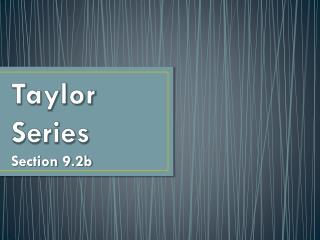DownloadDownload PresentationTaylor Series

# Taylor Series

Télécharger la présentation## Taylor Series

- - - - - - - - - - - - - - - - - - - - - - - - - - - E N D - - - - - - - - - - - - - - - - - - - - - - - - - - -
##### Presentation Transcript

1. Taylor Series Section 9.2b

2. Do Now Find the fourth order Taylor polynomial that approximates near Before finding a bunch of derivatives, remember that we can use a known power series to generate another… From the Table of Maclaurin Series on p.477: Therefore,

3. Do Now Find the fourth order Taylor polynomial that approximates near The Taylor polynomial: Support by graphing both functions in [–3, 3] by [–2, 2]

4. Practice Problems: #16 on p.478 Let f be a function that has derivatives of all orders for all real numbers. Assume Write the third order Taylor polynomial for f at x = 0 and use it to approximate f(0.2).

5. Practice Problems: #16 on p.478 Let f be a function that has derivatives of all orders for all real numbers. Assume (b) Write the second order Taylor polynomial for , the derivative of f, at x = 0 and use it to approximate . Second order Taylor polynomial for :

6. Practice Problems: #18 on p.478 The Maclaurin series for f(x) is (a) Find and In this case, Similarly,

7. Practice Problems: #18 on p.478 The Maclaurin series for f(x) is (b) Let g(x) = x f(x). Write the Maclaurin series for g(x), showing the first three nonzero terms and the general term. Multiply each term of f(x) by x: (c) Write g(x) in terms of a familiar function without using series.

8. Practice Problems: #20 on p.478 Let and Find the first four terms and the general term for the Maclaurin series generated by f. From the Table of Maclaurin Series on p.477: So factor out 2 and substitute for ...

9. Practice Problems: #20 on p.478 Let and Find the first four terms and the general term for the Maclaurin series generated by f.

10. Practice Problems: #20 on p.478 Let and (b) Find the first four nonzero terms and the Maclaurin series for G. The first term: To find the other terms, integrate the terms of the series for f:

11. Practice Problems: #20 on p.478 Let and (b) Find the first four nonzero terms and the Maclaurin series for G.